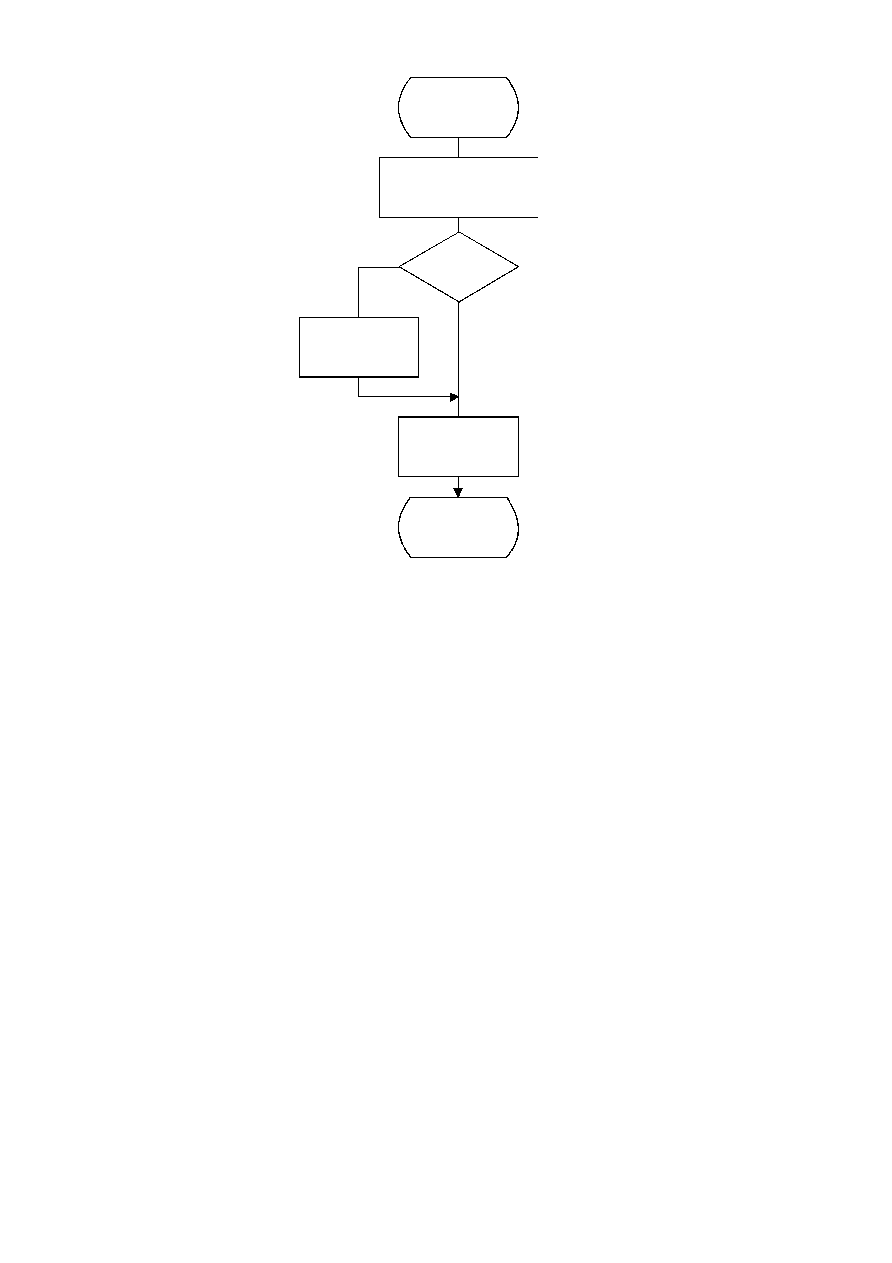ISO/IEC 10918-1 : 1993(E)
TISO1350-93/d073
Encode_R,ZZ(K)
SSSS = CSIZE(ZZ(K))
RS = (16 × R) + SSSS
Append EHUFSI(RS) bits
of EHUFCO(RS)
ZZ(K) < 0
?
Yes
No
ZZ(K) = ZZ(K) ­ 1
Append SSSS
low order bits of ZZ(K)
Done
Figure F.3 ­ Sequential encoding of a non-zero AC coefficient
Figure F.3 [D73] 14 cm = 547 %
Some of the procedures in Table D.1 are used in the higher level control structure for scans and restart intervals described
in Annex E. At the beginning of scans and restart intervals, the probability estimates used in the arithmetic coder are reset
to the standard initial value as part of the Initenc procedure which restarts the arithmetic coder. At the end of scans and
restart intervals, the Flush procedure is invoked to empty the code register before the next marker is appended.
F.1.4.1
Arithmetic encoding of DC coefficients
The basic structure of the decision sequence for encoding a DC difference value, DIFF, is shown in Figure F.4.
The context-index S0 and other context-indices used in the DC coding procedures are defined in Table F.4
(see F.1.4.4.1.3). A 0-decision is coded if the difference value is zero and a 1-decision is coded if the difference is not
zero. If the difference is not zero, the sign and magnitude are coded using the procedure Encode_V(S0), which is
described in F.1.4.3.1.
F.1.4.2
Arithmetic encoding of AC coefficients
The AC coefficients are coded in the order in which they occur in the zig-zag sequence ZZ(1,...,63). An end-of-block
(EOB) binary decision is coded before coding the first AC coefficient in ZZ, and after each non-zero coefficient. If the
EOB occurs, all remaining coefficients in ZZ are zero. Figure F.5 illustrates the decision sequence. The equivalent
procedure for the Huffman coder is found in Figure F.2.
CCITT Rec. T.81 (1992 E)
93